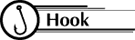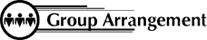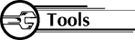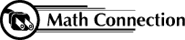8th Grade Math Home > Teacher Resources > Activities > Reflection: Student Worksheet

 Reflection: Student Worksheet Name: ____________________________________Look in the mirror. Raise your right hand. Does your reflection also raise its right hand?Students work individually.Each student needs: 3 sheets of graph paper 1 ruler color pencils protractors stencils (see attached)Trace the stencil on one side of the x-axis. Press hard with your pencil so your figure can be seen through a folded page. Now mark three points on the figure. Label them A, B, and C. Fold the first sheet of paper along the x-axis for a horizontal line of reflection. On the back of the graph paper trace the figure, including A, B, and C. Press hard with your pencil. Open the paper and trace that reflection on the front. Locate the images of A, B, and C in the reflected figure. Label the points A', B', and C'. Use a straightedge and a red pencil to connect A to A', B to B', and C to C'. Measure the angles where the line of reflection crosses each red segment. What do you observe? Mark the midpoint of each of the red segments. What do you observe? Find the coordinates of A, B, and C and A', B', and C'. What do you observe? Do numbers 1-8 using the y-axis as a vertical line of reflection. Do number 1-8 using the graph of y=x as a diagonal line of reflection.As a result of this activity, students will learn that some transformations, such as reflections and rotations, do not change the figure itself, only its position or orientation.

 8th Grade Math Home > Teacher Resources > Activities > Reflection: Student Worksheet